International
Tables for
Crystallography
Volume B
Reciprocal space
Edited by U. Shmueli

International Tables for Crystallography (2010). Vol. B, ch. 4.1, pp. 487-488   | 1 | 2 |

Section 4.1.3. Scattering of X-rays by thermal vibrations

B. T. M. Willisa*

aDepartment of Chemistry, Chemistry Research Laboratory, University of Oxford, Mansfield Road, Oxford OX1 3TA, England
Correspondence e-mail: bertram.willis@chem.ox.ac.uk

4.1.3. Scattering of X-rays by thermal vibrations

| top | pdf |

The change of frequency, or energy, of X-rays on being scattered by thermal waves is extremely small. The differential scattering cross section,, giving the probability that X-rays are scattered into the solid angleis thenwhereThe angled brackets indicate an average value over a period of time much longer than the period of oscillation of an atom. Q is the scattering vector' defined bywhere k and k0 are the wavevectors (each of magnitude) of the scattered and incident beams, respectively. For Bragg scattering the magnitude of Q is, whereis the Bragg angle.is the scattering factor of theth atom in the unit cell.

The cross sectioncan be expanded as a power series:The individual terms on the right-hand side refer to the cross sections for zero-order, first-order, second-order scattering …, i.e. for processes involving no exchange of energy between the incident radiation and the crystal (Bragg scattering), the exchange of one quantum of lattice vibrational energy (one-phonon scattering), the exchange of two quanta (two-phonon scattering) ….

The instantaneous thermal displacementof the atomcan be expressed, using equations (4.1.2.3), (4.1.2.6), (4.1.2.7) and (4.1.2.8), as a superposition of the displacements of the 3nN (∼1023) independent normal modes of vibration:Explicit expressions can now be given for the partial cross sections in equation (4.1.3.2). The cross section for Bragg scattering iswhere v is the cell volume. The delta function requires thatwhereis a reciprocal-lattice vector, so that scattering is restricted to the points h of the reciprocal lattice. The structure factor F(Q) iswhere the exponentof the temperature factor of the atomis calculated by summing over the normal modes:The last equation shows that the acoustic modes, with frequencies approaching zero as, make the largest contribution to the temperature factor.

The one-phonon cross section iswhereis the structure factor for one-phonon scattering' by the mode (jq) and is given byThe delta function in equation (4.1.3.6) implies thatso that the scattering from the 3n modes with the same wavevector q is restricted to pairs of points in reciprocal space which are displaced byfrom the reciprocal-lattice points. (These satellite reflections are analogous to the pairs of `ghosts' near the principal diffraction maxima in a grating ruled with a periodic error.) There is a huge number, N, of q vectors which are uniformly distributed throughout the Brillouin zone, and each of these vectors gives a cross section in accordance with equation (4.1.3.6). Thus the one-phonon TDS is spread throughout the whole of reciprocal space, rising to a maximum at the reciprocal-lattice points wherefor the acoustic modes.

For two-phonon scattering, involving modes with wavevectors q1 and q2, the scattering condition becomesThe intensity at any point in reciprocal space is now contributed by a very large number of pairs of elastic waves with wavevectors satisfying equation (4.1.3.8). These vectors span the entire Brillouin zone, and so the variation of the two-phonon intensity with location in reciprocal space is less pronounced than for one-phonon scattering.

Expressions forand for higher terms in equation (4.1.3.2) will not be given, but a rough estimate of their relative magnitudes can be derived by using the Einstein model of the crystal. All frequencies are the same,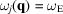, and for one atom per cell () the exponent of the temperature factor, equation (4.1.3.5), becomes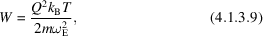assuming classical equipartition of energy between modes: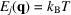. The cross sections for zero-order, first-order, second-order … scattering are then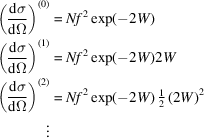and the total cross section is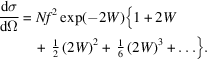The expression in curly brackets is the expansion of. The nth term in the expansion, associated with the nth-order (n-phonon) process, is proportional toor to. The higher-order processes are more important, therefore, at higher values ofand at higher temperatures.

Our treatment so far applies to the TDS from single crystals. It can be extended to cover the TDS from polycrystalline samples, but the calculations are more complicated as the first-order scattering at a fixed value ofis contributed by phonon wavevectors extending over the whole of the Brillouin zone. For a fuller discussion of the TDS from powders see Section 7.4.2in IT C (2004).

References

International Tables for Crystallography (2004). Vol. C. Mathematical, Physical and Chemical Tables, edited by E. Prince. Dordrecht: Kluwer Academic Publishers.